# Circuit Flashcards Preview

## FE > Circuit > Flashcards

Flashcards in Circuit Deck (17):
1

## Thevinen Equivalent voltage2

## Two parallel flat plates are separated by 2cm. A voltage of 500 V is applied to the parallel plates. What is the maximum electric field force experienced by an electron passing between the plates?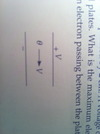3

## the rms current through the 45-ohm resistor in the circuit shown is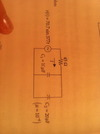4

## the current in the 100 ohm resistor is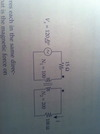4

5

## the instantaneous current through the inductor at t=0.001 sec in amperes is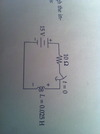6

7

8

9

## the rms voltage across the 40-ohm resistor is, in volts:10

11

12

13

14

## Two parallel conductors carry 50 amperes each in the same direction. The conductors are 5 cm apart. What is the magnetic force on 1 meter length of one conductor in newtons?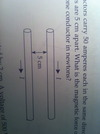15

16

## The power delivered by the 12 volt battery, above, in watts, is: